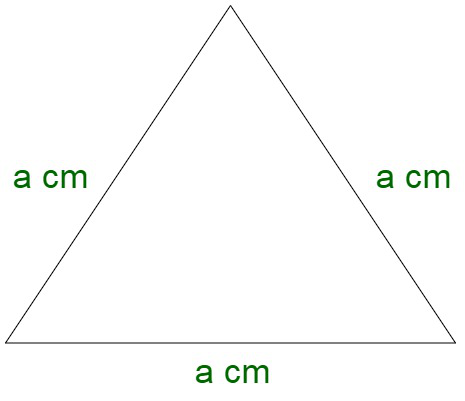# What is the relation between the perimeter and the side length of an equilateral triangle?

• Last Updated : 09 Nov, 2021

An equilateral triangle is a two-dimensional figure, where all the angles and the sides are exactly equal to each other. It is also known as the equiangular triangle since all of its angles are equivalent to 60° each. It is a regular polygon composed of three equal sides.Properties of an equilateral triangle

• All three sides are equal.
• All the three angles are equal i.e 60° each.
• Median, angle bisector, and altitude are all equal in an equilateral triangle.
• The area is given by √3/4(a)square.
• All angles are congruent to each other.

### Perimeter

The perimeter of an equilateral triangle is given by the sum length of all the sides of this figure. Since an equilateral triangle is composed using three equal sides, the perimeter is given by the summation of all these sides.

Let us assume the side of the equilateral triangle to be a.

Now, the perimeter P is given by,

P = a + a + a

P = 3a

Therefore, the perimeter of an equilateral triangle is equivalent to thrice the side length of the triangle.

### Sample Questions

Question 1. If the side of an equilateral triangle is 2√3. Then find the perimeter of the equilateral triangle?

Solution:

Here we have to find the perimeter of the equilateral triangle

We are given that the side of an equilateral triangle is 2√3

As we know that

Perimeter of an equilateral triangle = Side + Side + Side

Perimeter of an equilateral triangle = 2√3 + 2√3 +2√3

Perimeter of an equilateral triangle = (2 + 2 + 2)√3

Perimeter of an equilateral triangle = 6√3

Question 2. Assume that the perimeter of an equilateral triangular park is 543m, then find the sides of the equilateral triangular park?

Solution:

Here we have to find the sides of the equilateral triangular park using its perimeter

Given,

The perimeter of equilateral triangular park = 543 m

As we know that

Perimeter of equilateral triangular park = Side + Side + Side = a + a + a = 3a

Perimeter of equilateral triangular park = 543 m = 3a

543 = 3a

a =a = 181 m

Therefore,

Side of the equilateral triangular park is 181 m.

Question 3. Find the cost of fencing triangular school playground if all the sides of triangular school playground are equal that is 50 m. If the cost of fencing per meter is 23.

Solution:

Here we have to find the cost of fencing of triangular school playground

For that first, we have to find the perimeter of the playground

As we know that

Perimeter of triangle = side + side + side

Therefore,

Perimeter of triangular school playground = 50 + 50 + 50

Perimeter of triangular school playground = 150 m

Now we will find the cost of fencing the playground

According to the question

Cost of fencing per meter is ₹23

Therefore,

Cost of fencing 150 m = 23 × 150 = ₹3450

Hence,

The cost of fencing the school playground is ₹3450.

Question 4. Perimeter of equilateral triangular signboard hoarding is 450 cm. Find the area of the sign hoarding?

Solution:

Here we have to find the area of the triangular sign hoarding

For that first, we need to find the side of the equilateral triangular sign hoarding

According to the question

The perimeter of the triangular sign hoarding = 450 cm

As we know that

Perimeter of the triangular sign hoarding = Side + Side + Side = 3a

3a = 450

a =a = 150 cm

Further finding the area of sign board

Area of equilateral triangle =Area of equilateral triangle =Area of equilateral triangle = 5625√3

Question 5. If the side of an equilateral triangle is 20√5. Then find the perimeter of the equilateral triangle?

Solution:

Here we have to find the perimeter of the equilateral triangle

We are given that the side of an equilateral triangle is 20√5

As we know that

Perimeter of an equilateral triangle = Side + Side + Side

Perimeter of an equilateral triangle = 20√5 + 20√5 +20√5

Perimeter of an equilateral triangle = (20 + 20 + 20)√5

Perimeter of an equilateral triangle = 60√5

My Personal Notes arrow_drop_up